An Introduction to Thermal Analysis of Thermosets

The current experiment was performed to find the glass transition temperature using a method which depends upon the change of elastic behavior.

Sample

The sample used was cured KU600 epoxy resin powder in the form of an adhesive layer between two plates of metal.

Conditions

Measuring cell: TMA

Probe: Ballpoint probe of quartz glass of 3 mm diameter, and a 3-point bending accessory.

Sample Preparation

The KU600 epoxy resin powder was cured between two steel plates of 0.1 mm thickness, which were razor blade pieces 16mm by 5 mm in size. The resin powder was 0.06 mm thick. The effect was to produce a sandwich structure as is seen with adhesive bonding. The cured sample was then mounted on the three-point bending accessory part to keep the full 14 mm length between the supports.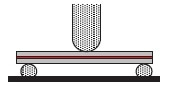TMA Measurement

The sample was heated from 50 oC to 240 oC at 5 K/min, with the load alternating between 0.5 N and 1.0 N at intervals of 6 seconds, for a period of 12 seconds.

Atmosphere

Static air was used as the atmosphere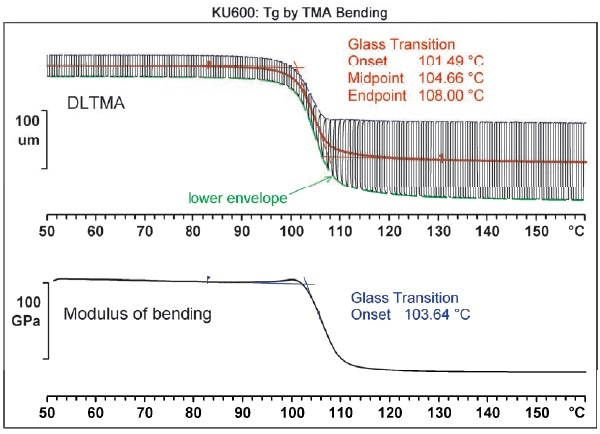Interpretation

The sandwich structure bends slightly due to the force applied to the probe. When there was a 0.5 N change in force the change in bending was about 40 µm, and lasted as long as the cured epoxy powder remained below the glass transition temperature. Once above this temperature, the bending became more obvious to about 200 µm at 1 N.

There is also a more pronounced change in bending as the force changes, to about 130 µm. The DLTMA curve records this change and thus the glass transition or softening temperature can be found. This is evaluated as either the midpoint or onset of the mean curve represented by the red line.

The dashed lines denote the upper and lower envelopes which represent the bending profiles for the forces on the probe at 0.5 N and 1.0 N. the difference between them corresponds to the elasticity for the modulus of bending. The assumption is that the elastic modulus of the steel pieces changes very little, and thus the detected changes are due to the epoxy thermoset material. The curve shown by the bending modulus of the sandwich is also a measurement used to find the glass transition temperature of the epoxy adhesive, as the onset in the lower diagram shows.

Evaluation

It is possible to calculate the bending modulus E of the sandwich from the DLTMA curve directly using the following formula: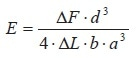Where ΔF represents the change of the force on the probe

ΔL is the corresponding change in bending

Since the geometry of the sample is determined by the thickness (a), the width (b) and the distance between the supports (c), ΔL represents the distance between the envelopes. Thus the amplitude of the DLTMA is in reciprocal relation to the modulus. A blank curve must be subtracted to find the bending modulus.

The static bending is used to determine the softening temperature. For instance, the mean value curve or an envelope is used similar to a penetration measurement. It is therefore possible to find the softening temperature from a measurement which does not have an alternating load, as at a constant IN force. In this situation, the curve would correspond roughly to the lower of the two envelopes.

The glass transition temperature is typically calculated from the bending modulus curve at its onset, as is done in DMA tests.

Table 1. Glass transition temperatures from DLTMA.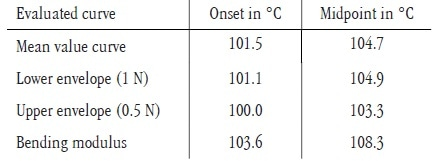In Table 1, the results of multiple evaluations are given in summary, showing the effect of the evaluation on the determination of glass transition temperatures as found by the TMA method. The temperatures depend on the frequency as shown by the DMA measurement results.

Conclusions

Using bending measurements with both static and changing force on the probe provides a sensitive method to find the softening or glass transition temperature of adhesive bonding layers, coatings or filled thermosets. The point of study here is the temperature at which the change of bending or modulus occurs in the sample.

Thus multiple measurements are avoided and the overall test time is reduced. Sample preparation is simplified since the sample geometry only needs to be fitted to the bending measurement accessory. The fact that only limited force can be applied in the TMA even at maximum means that bending samples need to be extremely thin, allowing the measurement of even minimal bending and change in bending.

When the results of bending measurements are reported, the method used and the mode of evaluation should be mentioned as well.

Other than DMA, the DLTMA measurement is in many cases the only way to find the softening or glass transition temperature of a highly filled or highly reinforced thermoset material. This is because these materials have very minute changes in heat capacity and expansion coefficient at the glass transition temperature.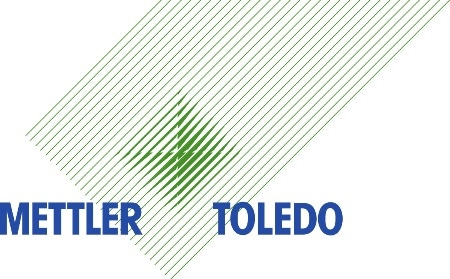This information has been sourced, reviewed and adapted from materials provided by Mettler Toledo - Thermal Analysis.

For more information on this source, please visit Mettler Toledo - Thermal Analysis.

Citations

Please use one of the following formats to cite this article in your essay, paper or report:

• APA

Mettler Toledo - Thermal Analysis. (2019, July 25). An Introduction to Thermal Analysis of Thermosets. AZoM. Retrieved on October 16, 2019 from https://www.azom.com/article.aspx?ArticleID=16411.

• MLA

Mettler Toledo - Thermal Analysis. "An Introduction to Thermal Analysis of Thermosets". AZoM. 16 October 2019. <https://www.azom.com/article.aspx?ArticleID=16411>.

• Chicago

Mettler Toledo - Thermal Analysis. "An Introduction to Thermal Analysis of Thermosets". AZoM. https://www.azom.com/article.aspx?ArticleID=16411. (accessed October 16, 2019).

• Harvard

Mettler Toledo - Thermal Analysis. 2019. An Introduction to Thermal Analysis of Thermosets. AZoM, viewed 16 October 2019, https://www.azom.com/article.aspx?ArticleID=16411.

Tell Us What You Think

Do you have a review, update or anything you would like to add to this article?